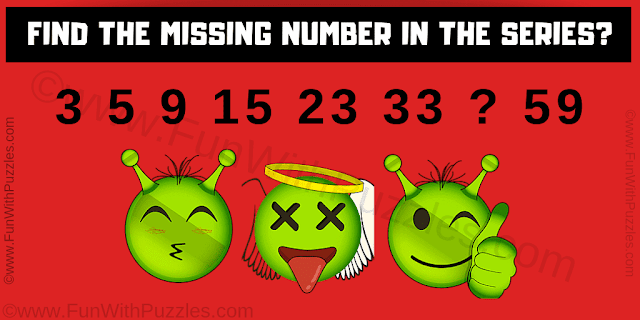## Thursday, May 9, 2019

This is the simple number series question for school going students to test your maths skills. In this number series question, you are shown some numbers in a sequence. Your challenge is to first find the logical pattern used in this number series and then find the value of the missing number which will replace the question mark in this series.Can you find the missing number in this series?
The answer to this "Number Series Question", can be viewed by clicking on the answer button.

1.i thought 45 is the answer. how it is 26?

1.Yes, you are right. The answer to this number series #question should be 45. This answer to this #puzzle will be updated soon.

2.Answer and logical reasoning explanation to this #puzzle is updated to 45 now.

A comment doesn't cost a thing. Please drop a comment below to boost the author's morale.